## Gradient accumulation and GPU memory

• We have explored the simplest neural net with fully connected linear layers in earlier lectures. In this lecture we will focus on tweaking first and last layers, in the next few weeks on tweaking middle part of the neuralnet.
• Review of the notebook Road to Top part 2 and congrats to fastai students beat Jeremy on 1st and 2nd
• What are the benefits of using larger models? What are the problems of larger models? (use up GPU memory as GPU is not as clever as CPU to find ways to free itself; so large model needs very expensive GPU) What can we do about it when GPU out of memory? first, to restart the notebook; then Jeremy is about to show us a trick to enable us to train extra large models on Kaggle, Wow!
• How big is Kaggle GPU? Do you have to run notebooks on kaggle sometimes for example code competitions? Why it is good and fair to use Kaggle notebook to win leaderboard?
• How did Jeremy use a 24G GPU to find out what can a 16G GPU do? How did Jeremy find out how much GPU memory will a model use? How did Jeremy choose the smallest subgroup of images as the training set? Will training the model longer take up more memory? (No) So, smallest training set + 1 epoch training can quickly tell us how much memory is needed for the model.
• Jeremy then trained different models to see how much memories they used up. How much memory does convnext-small model take? Which line of code does Jeremy use to find out the GPU memory used up by the model? Which two lines of code does Jeremy use to free unnecssarily occupied memories GPU so that you don’t need to restart the kernel to run the next model?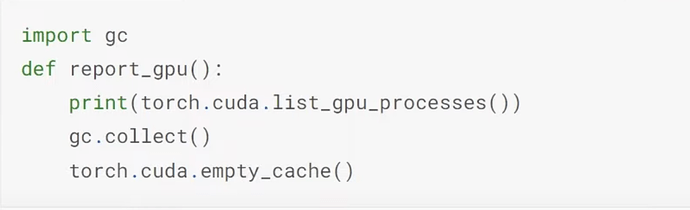• What if a model causes a crash problem of cuda out of memory? What is GradientAccumulation? What is integer divide? (`//`).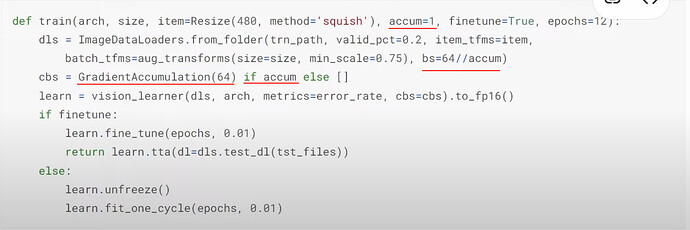• What is the problem of using smaller batch size? (smaller batch size, larger volatility of learning rate and weights) How can we make the model train in smaller batch size as if it is in large batch size? How to explain GradientAccumulation in code?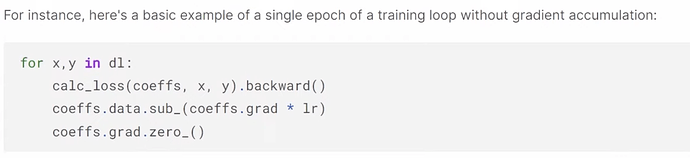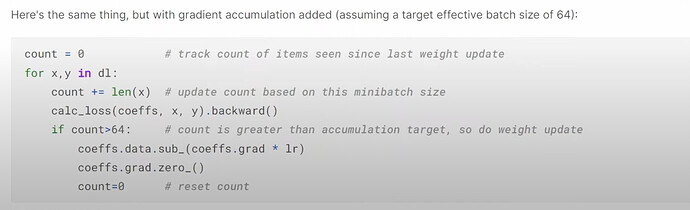• What is the implication of using GradientAccumulation? How much difference is the numeric result between using GradientAccumulation and not? What is the main cause for the difference?
• More questions: it should be `count &gt;= 64` in the code above when doing GradientAccumulation; `lr_find` uses batch size from the DataLoader;
• Why not just use a smaller batch size instead of GradientAccumulation? What is the rule of thumb for picking batch sizes? How about adjusting learning rate according to the batch size?
• How did Jeremy use GradientAccumulation to find out how many `accum` is needed to run those large models on Kaggle’s 16G GPUs? (`accum=1` always out of memory, but `accum=2` works for all large models).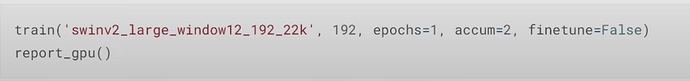## Creating an ensemble

• How did Jeremy put all the models and their settings together for experimenting later? Do we have to use the size of the model’s specification for now and how about in the future?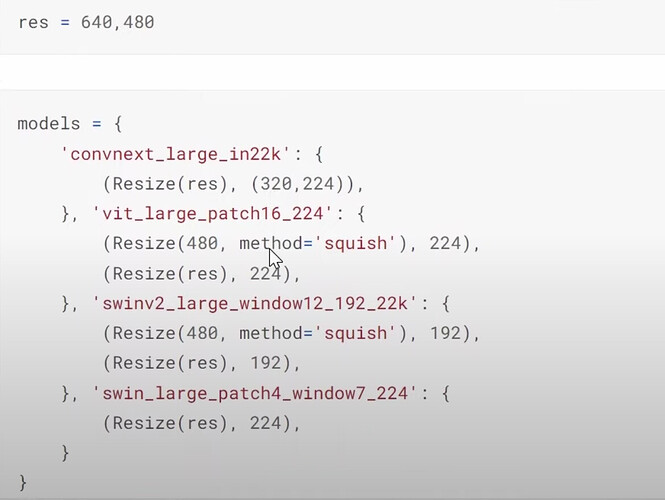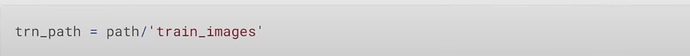• How to run all the models with specifications without running out of memory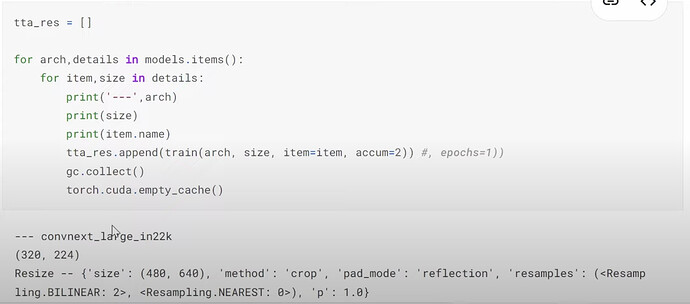• Why does Jeremy don’t use `seed=42` here in training? What is the effect?
• What is ensemble or bagging of different good deep learning architectures? Why it is useful?
• How to do the ensemble of different deep learning models?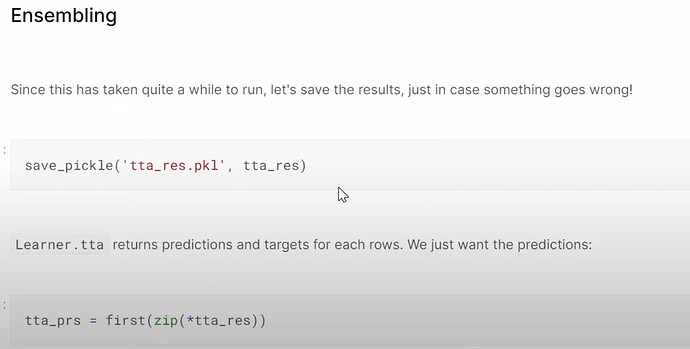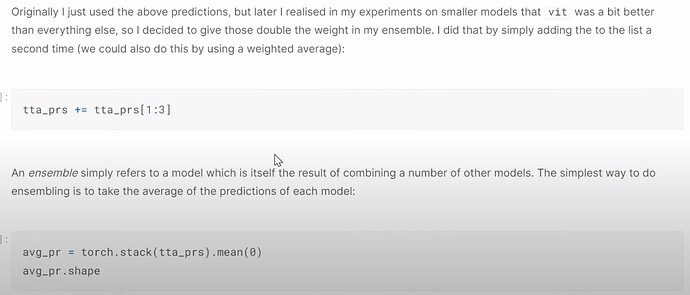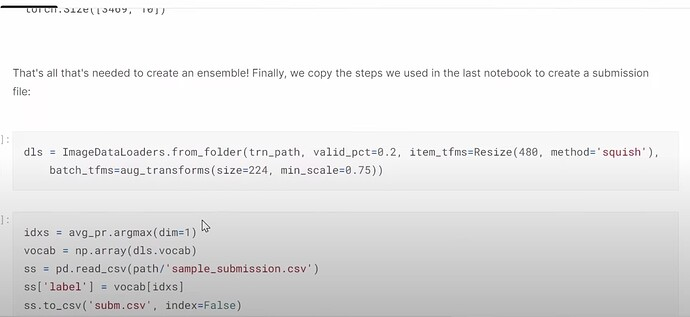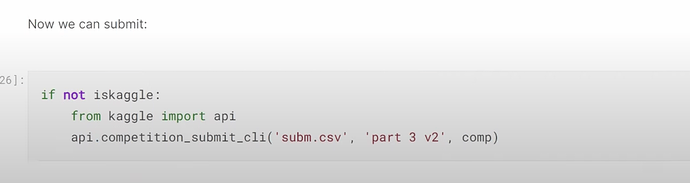• Why should we improve and submit to Kaggle everyday? How the submission history can help trace your models developments and improvement?
• More questions: What is k-fold cross-validation and how can it be applied in this case? Why does Jeremy don’t use it?
• Are there any drawbacks of GradientAccumulation? Any GPU recommendations?
• In part 2 Jeremy may cover how to train a smaller model to do well as in large models for faster inference

## Multi-target model

• How to set the data split and item and batch transformations?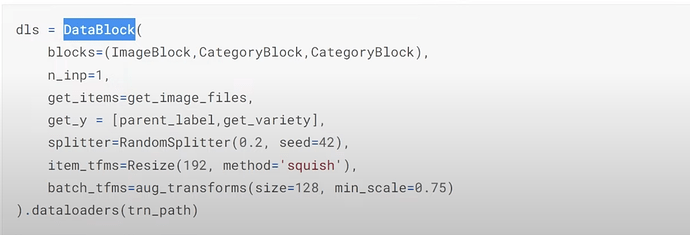• How to create a model to predict both disease and variety types? Can we see predicting both disease and variety in terms of predicting 20 things, 10 for disease, 10 for variety?
• What does the new model (and new dataloaders) need now to make predictions on disease?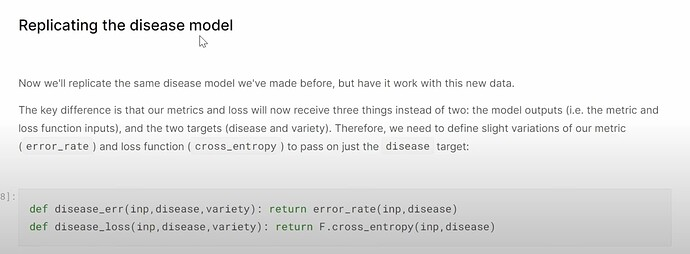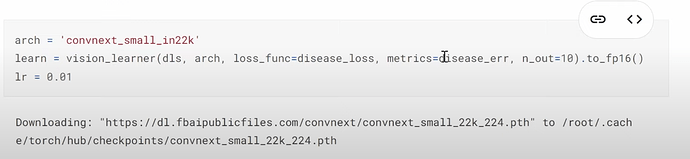• When and how to provide our own loss function? fastai can detect appropriate loss for your datalaoders and use it by default in simple cases. In this special case, How do we create and use our custom loss for the new model?

## Cross-entropy and softmax

• What does `F.cross_entropy` do exactly? This function belong to the first and last layer, therefore we must understand them. What is the raw output of the model of predicting 5 things?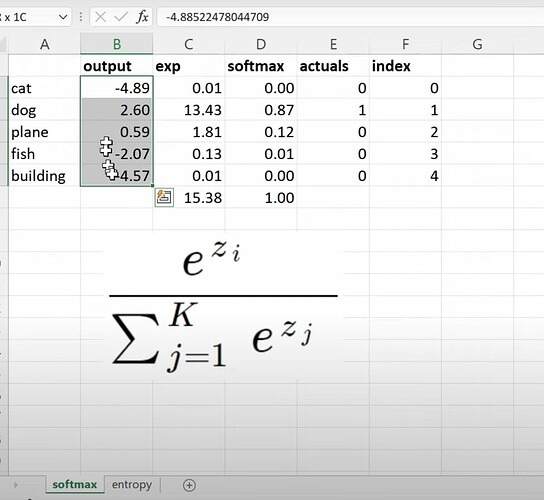• What is the formula of softmax and How to calculate it in the spreadsheet?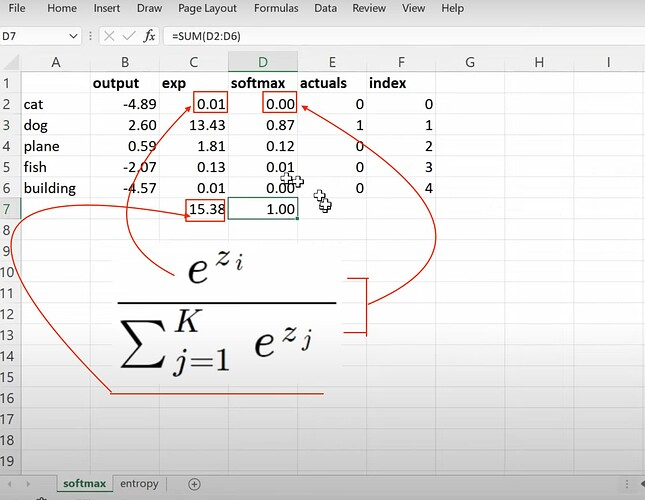• What is the problem of softmax? How does it make the obvious wrong prediction when given a cat image to the bear classifier?
• What can we do about the problem of the softmax above? (all prediction probabilities not adding up to 1). When do you use softmax and when not to?
• What is the first part of the cross_entropy loss formula?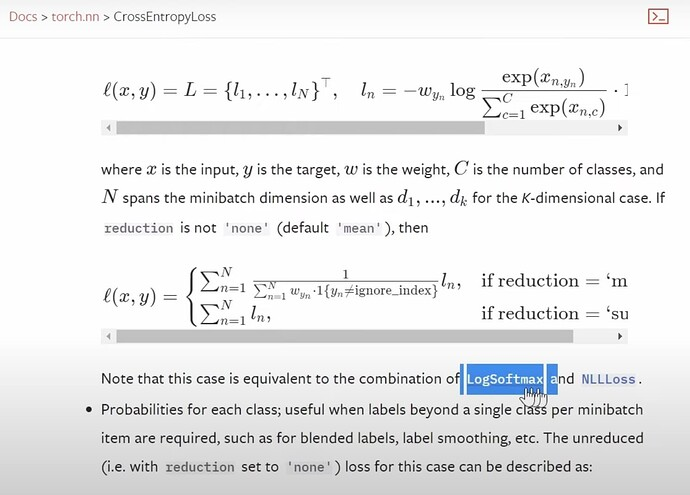• How to calculate cross-entropy from softmax?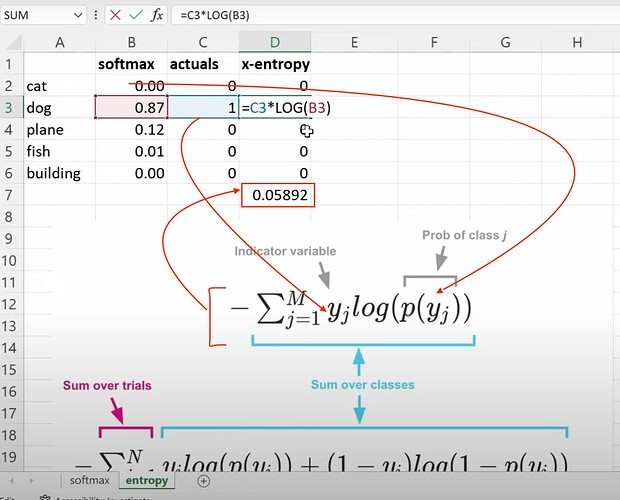• How to calculate binary-cross-entropy? How to understand its formula in predicting whether it is a cat or non-cat image? How to finally get the binary cross-entropy loss of a batch of 5 images?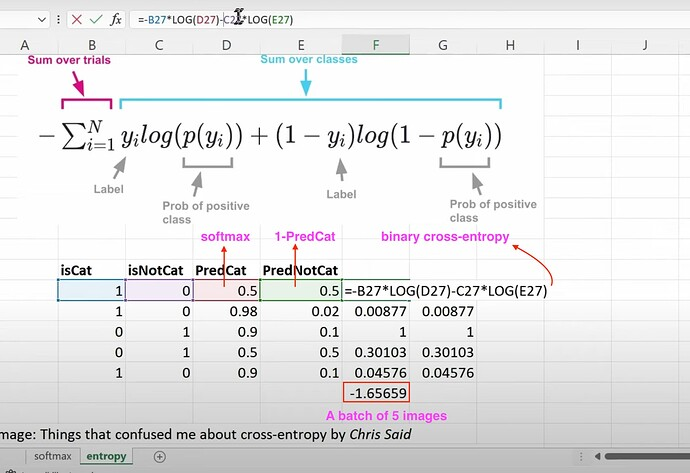• What are two versions of cross-entropy in pytorch? and when to use each version? Which version do we use here?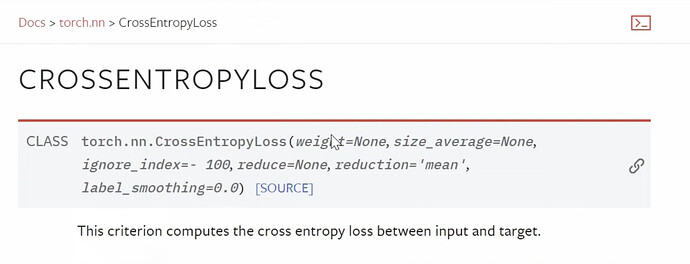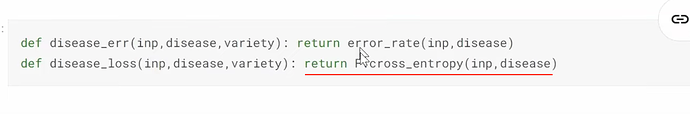## Multi-target activations

• With a dataloader having two targets, our new model needs to be informed what exactly is the loss func, metrics, and the size of output?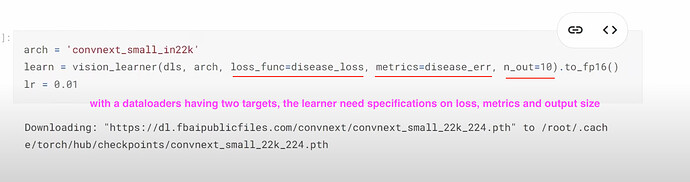• How to create a learner for prediction two targets or 20 items? How does a learner use disease and variety losses to know which 10 items are disease predictions and which 10 are variety predictions? How to combine two loss functions together? How to understand the combined loss?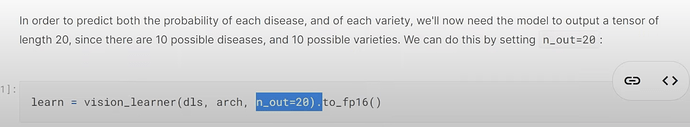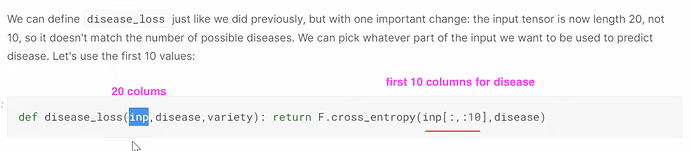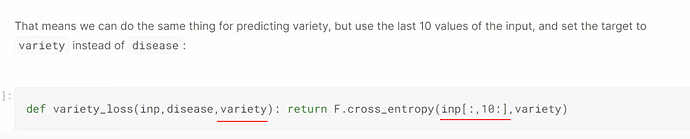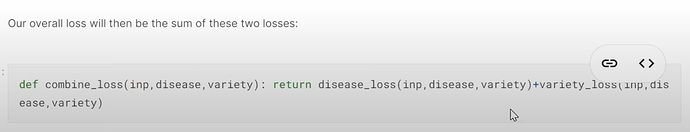• How to calc error rate for disease types and variety types? How to put them together and display them during training?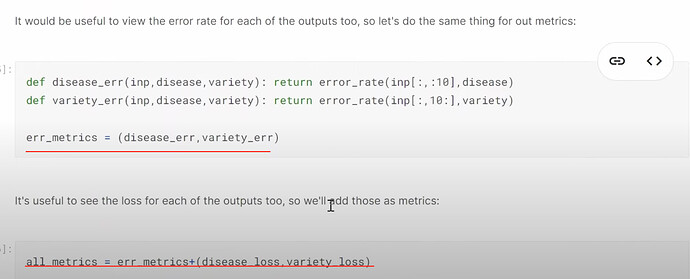• How to make the new learner and how did it train? Why the multi-task model didn’t improve and even a little worse than the previous model? Why training the multi-task model longer could improve the accuracy on disease prediction? Why predicting a second thing together could help improve the prediction of the first thing? Using multi-task model did improve the result in a Kaggle fish prediction competition Jeremy did before. What are the reasons or benefits for building multi-task models?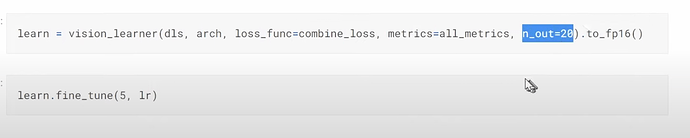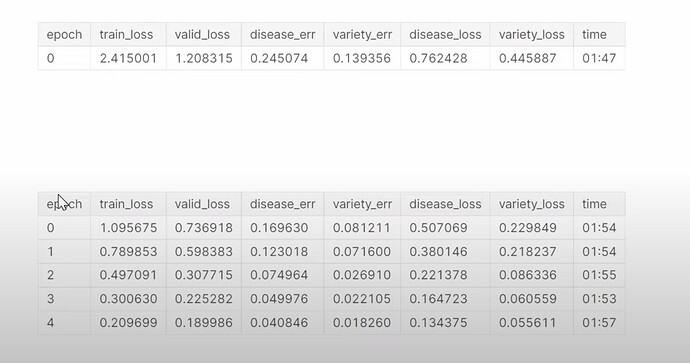How to make multi-task modeling less confusing to you? (build a multi-task for Titanic dataset from scratch; explore and experiment this notebook) by Chris Said of binary-cross-entropy?

## Collaborative filtering

• Collaborative filtering deep dive as chp 8 without change. What is the dataset used? Which version of the data we are using? How to read a tsv file using pandas? How to read/understand the dataset content/columns? recommendation system industry and Radek. How does Jeremy prefer to see the data? (cross tabulated) Why the image Jeremy talking about his preferred way of seeing the data has so few empty or missing data?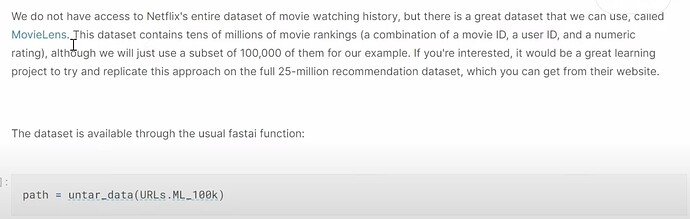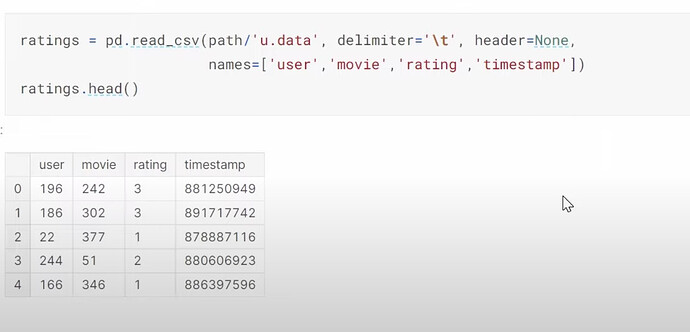• How to fill in the missing data or gaps in the cross tabulated dataset? How to figure out whether a new user would like a particular movie which he/she has not watched before? Can we figure out what kind/genre of movie is the particular movie we are talking here? What does the type probabilities of a movie look like? What does a user’s preference probabilities look like? If we match the two sets of probabilities up, can we know how much does the user like the movie? How do we calculate that?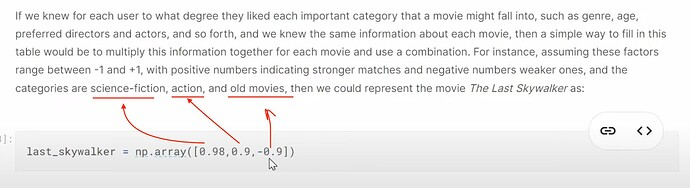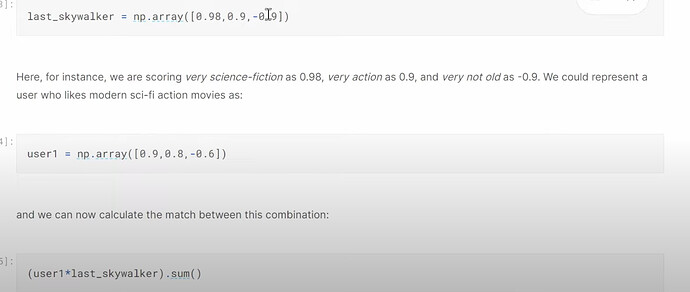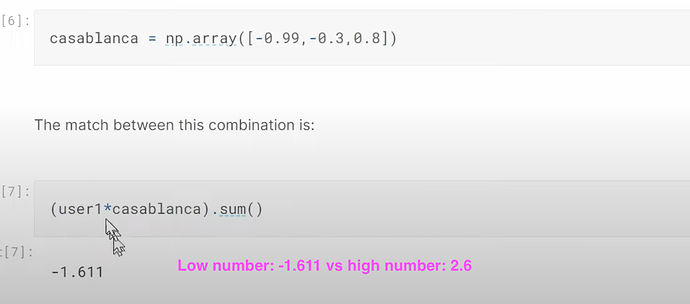• So far so good, what is the problem of the approach of doing dot product between user preference probabilities and movie type probabilities to find out our new user’s rating of the movie? (we don’t know neither of the probabilities). How are we going to deal with this problem? Can we create such movie type probabilities without knowing even the types?
• What is the latent factors? If I don’t know anything about the movies, can we use SGD (stochastic gradient descent) to find them? Can we create a random 5 numbers as a movie’s 5 latent factors for describing the types of the movie, and figure them out later? Can we create latent factors for each user too? Now how to calc the probability of a user likes a movie? (mmult or dot product between two groups of latent factors).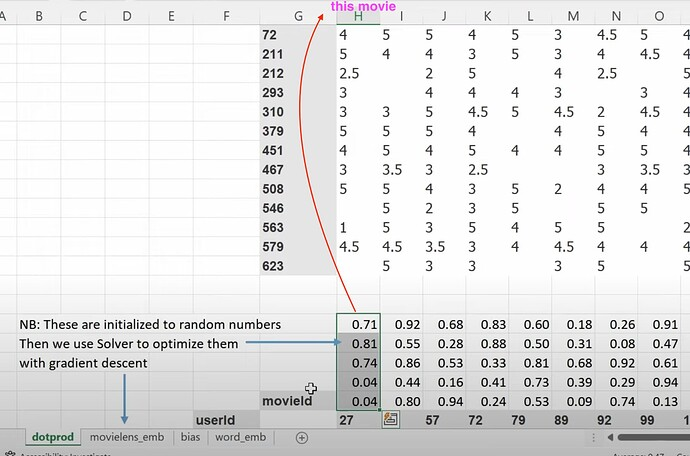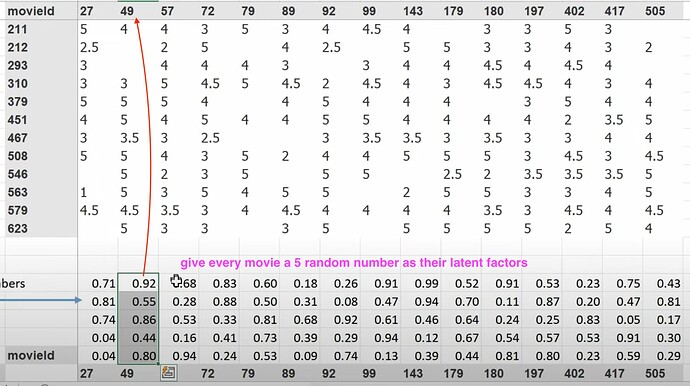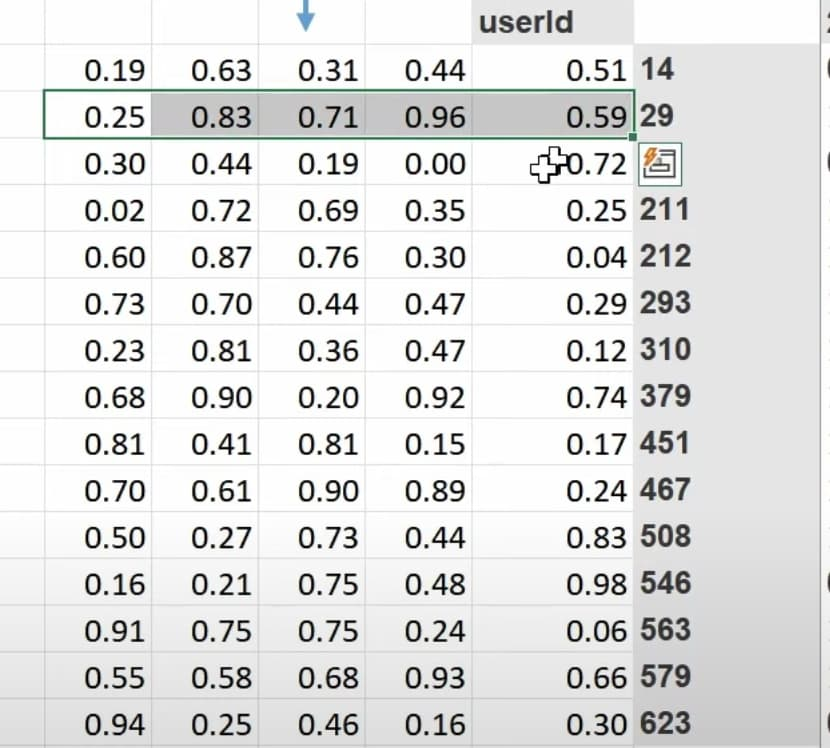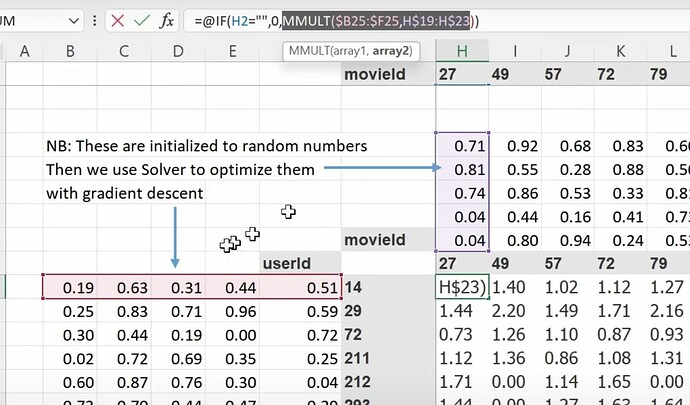• Now the mmult or dot product can give us the prediction of how much a user likes a movie, so we can compare predictions with true label. What to do when there is a missing label or data? (we make the prediction empty or zero). Can we use SGD to improve the latent factors by comparing predictions with labels using a loss function? How to use excel solver to update latent factors using SGD and the loss?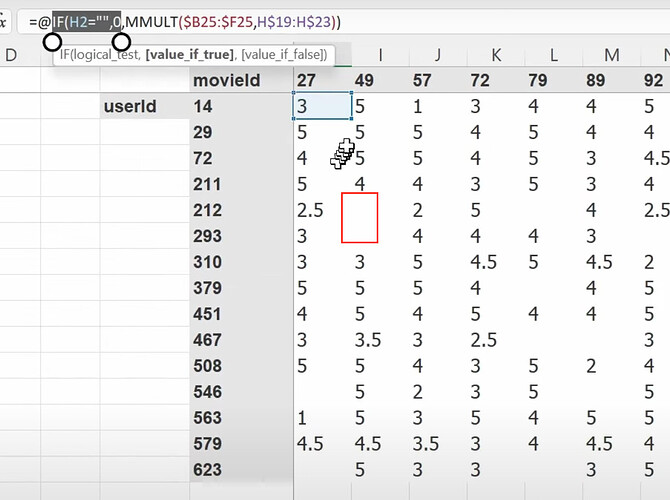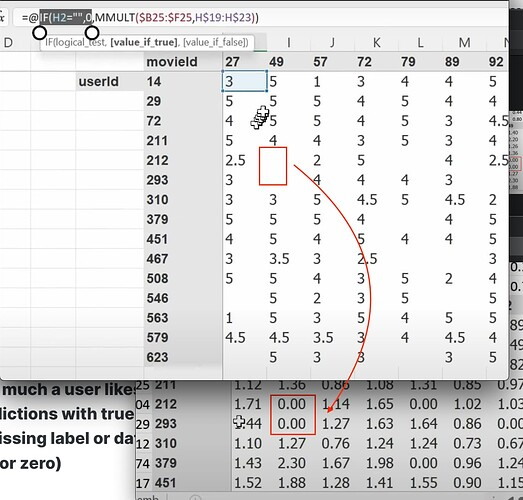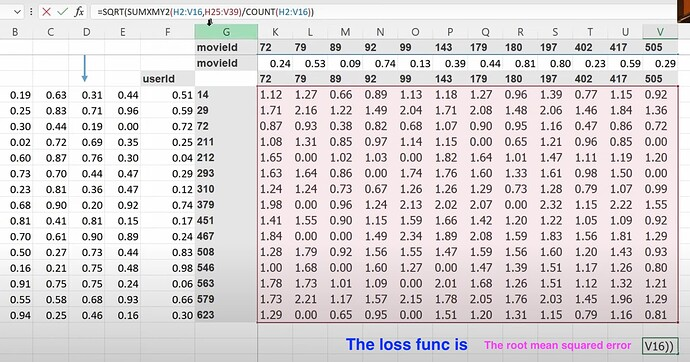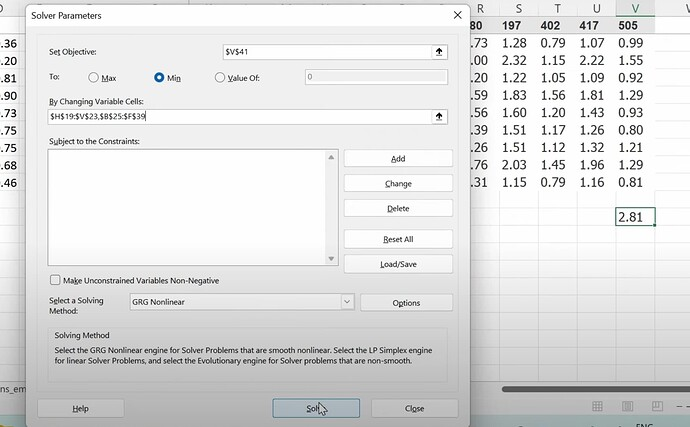Why excel is so slow on calc gradients with even small dataset? What is the basis of collaborative filtering? (if we know A likes (a, b, c) and B likes (a, b, c), then if A likes (d, e), maybe B likes (d, e) too). - Is the cosine of an angle between two vectors is the same thing as the dot product? - How do we do the things above in pytorch as they have different data format from excel? What does the dataset would look like in pytorch?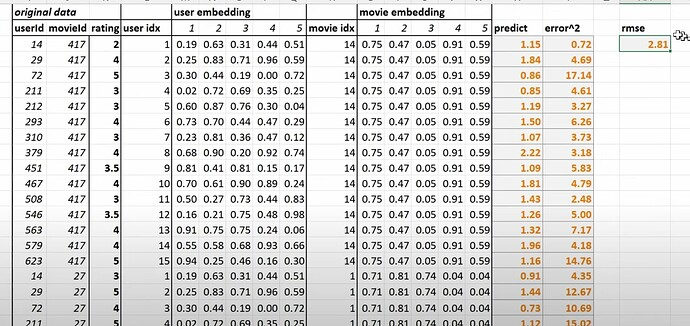## Embeddings

• What is embedding? What are embedding matrix, user embeddings, and movie embeddings? (embeddings = look up something in an array). The more intimidating words created in a field, the less intimidating the field actually is.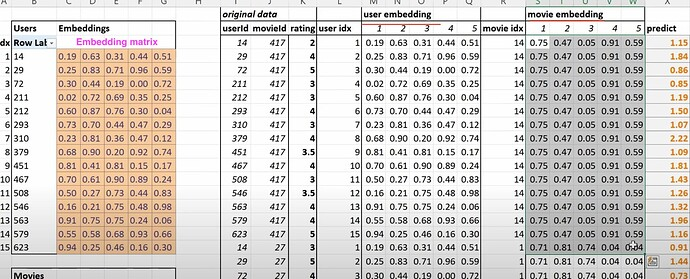• What does our dataset look like before building a dataloaders on it? How to create a dataloaders for collaborative filtering using `CollabDataloaders.from_df`? What does its `show_batch` look like? How do we create the user and movie latent factors algetother?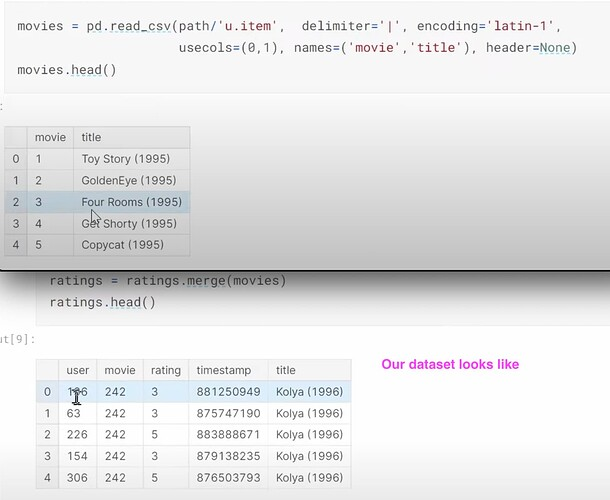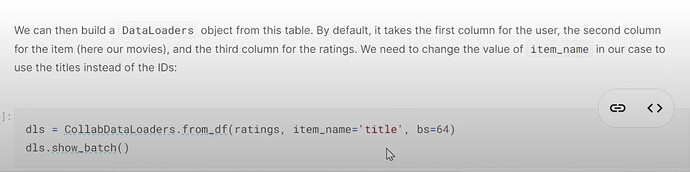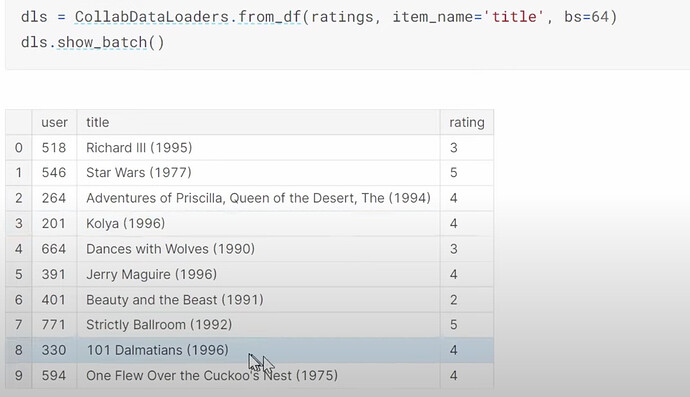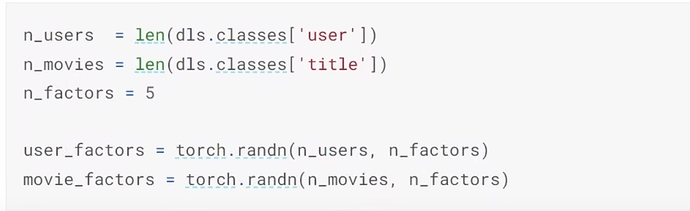• How do you choose the number of latent factors in fastai?
• How to understand looking up in excel for latent factors and doing dot product with one-hot embeddings are actually the same thing? Can we think of embeddings as a computational shortcut to multiply something by a one-hot-encoded vector? Can we think of embedding as a cook math trick of speeding up the matrix multiplication with dummy variables (without creating dummy variables nor one-hot encoded vector).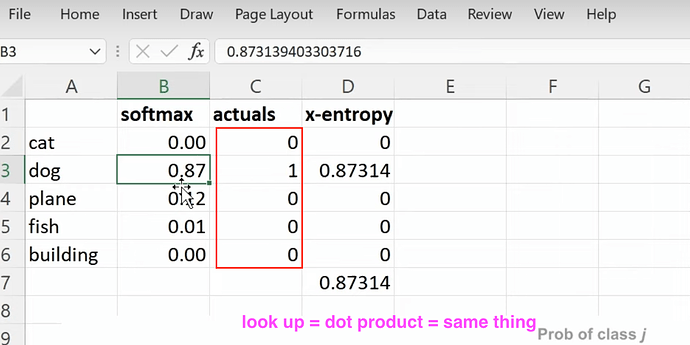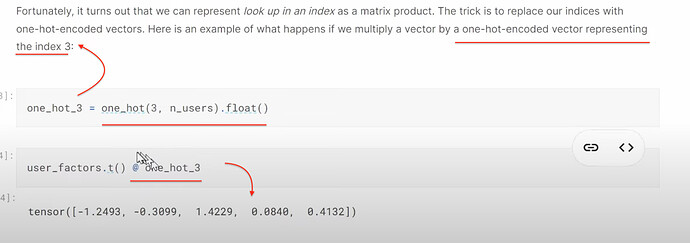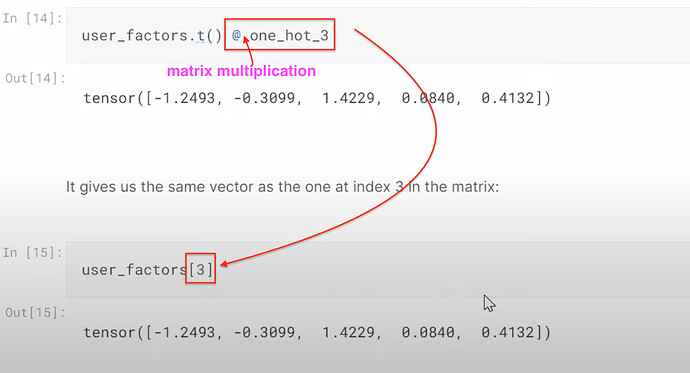## Object oriented programming

• How to build a collaborative filtering model from scratch? How do we create a class? (as a model is a class). How do we initiate a class object by `__init__`? Does `__init__` tell us what parameters to give in order to create a class instance? How does the class function `say` do? What is a super class? Where do we put it when creating a class? What does it give us? What is the super class (Module) for pytorch and fastai to use when creating a class? What does the `DotProduct` class look like?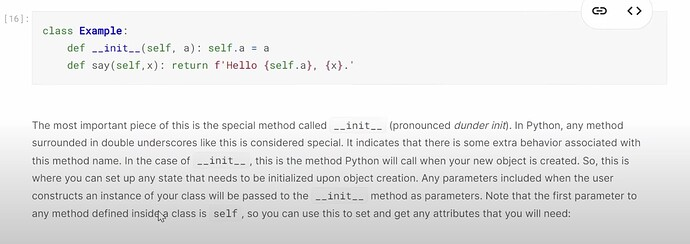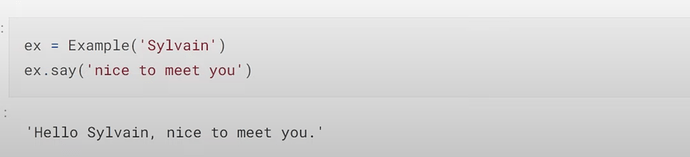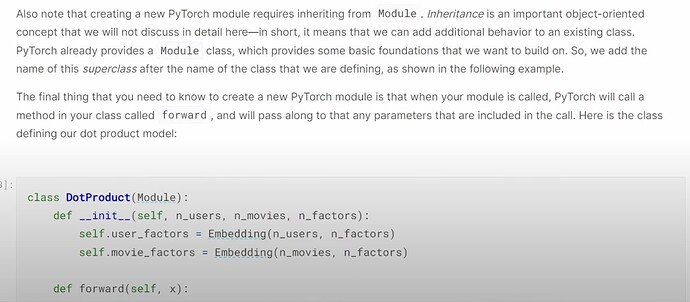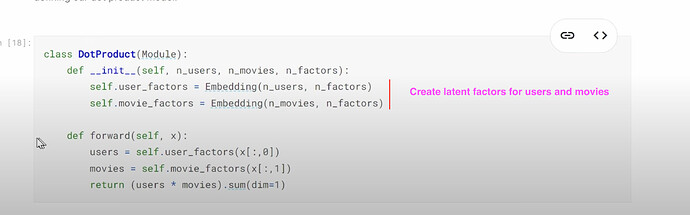• How to understand the `forward` function in the `DotProduct` class? What does `.sum(dim=1)` mean? (sum each row).## Improving collaborative filtering

• How to create a collab learner and start training? The training is very fast even on CPU.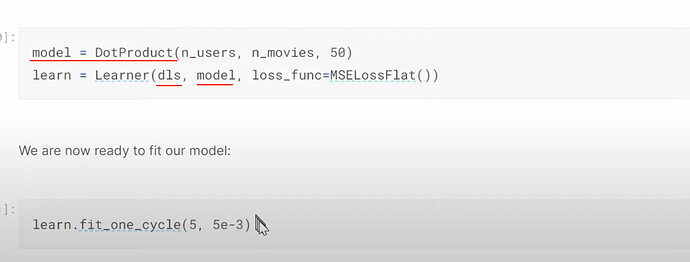• Why this collab model above is not great? (people who give ratings are people who love movies, they don’t rarely give 1, but many high ratings. Whereas the predictions have many occassions with ratings over 5). Review the sigmoid usage. How can we do sigmoid transformation to the predictions? How does this sigmoid work? Why do we use the up limit of the range `5.5` instead of `5`? Does adding sigmoid always improve the result?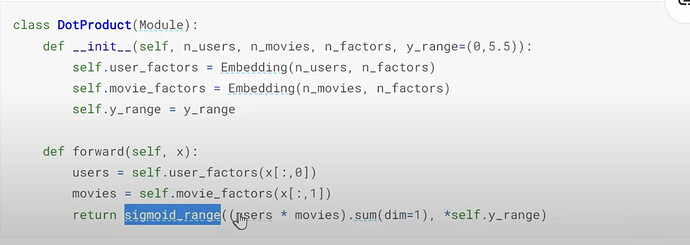• What interesting things did Jeremy observe from the dataset? (some users like to give high ratings to all movies, some tend to dislike all movies). Can we add one bias value to both user and movie latent factors to explain this interesting observation? How to use the bias factors inside the collab model?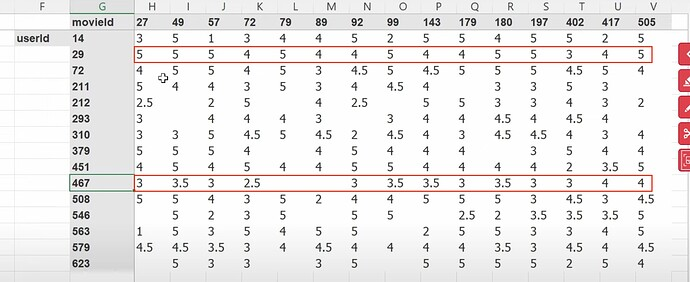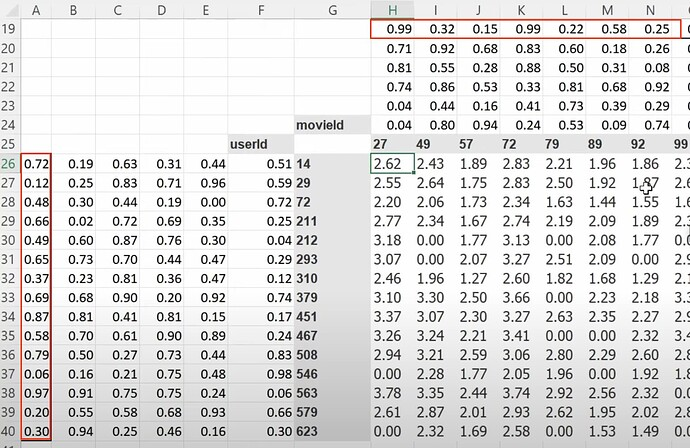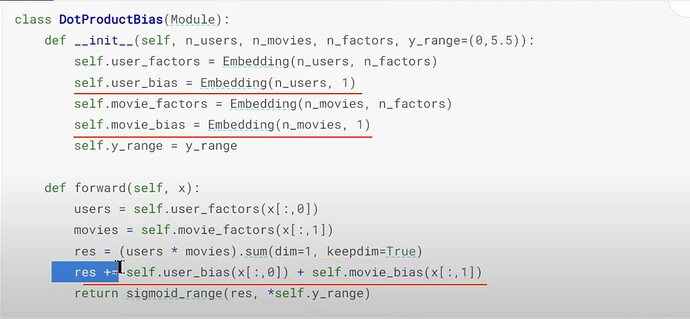• Why did the upgraded model with bias get worse? (overfitting).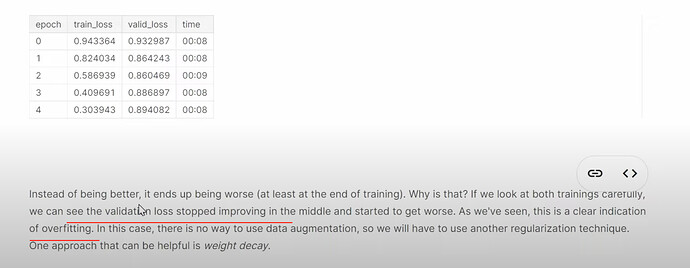## Weight decay

• What is weight decay and How does it help? How to understand weight decay in solving the problem of overfitting?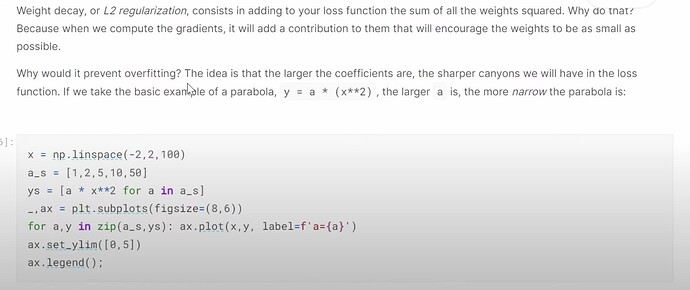• How to actually use weight decay in fastai code? Does fastai have a good default for collaborative filtering like CV? How does Jeremy suggest to find the appropriate `wd` value for your own dataset?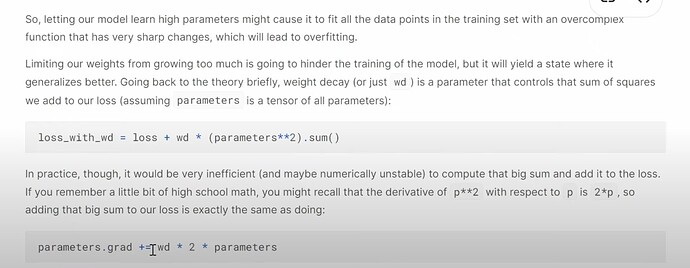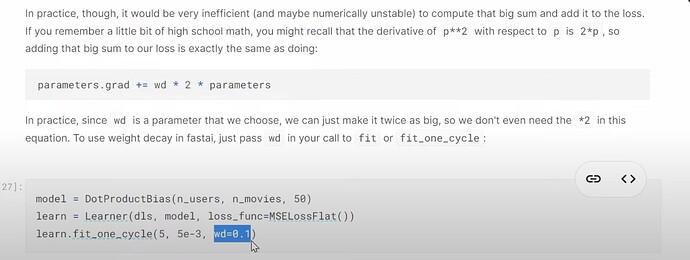• What is regularization? What’s wrong when the weights having high values or low values? How does weight decay help balance?
• More questions: any other rules other than Jeremy’s rule of thumb on number of latent factors, and recommendation on average rating is viable only when there are many metadata.